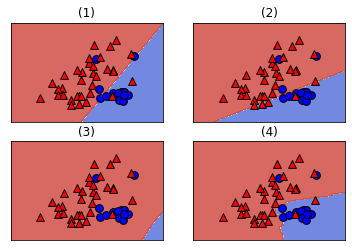## Scikit-learn refresher

### KNN classification

this exercise you'll explore a subset of the Large Movie Review Dataset.The X variables contain features based on the words in the movie reviews, and the y variables contain labels for whether the review sentiment is positive (+1) or negative (-1).

Large Movie Review Dataset :This is a dataset for binary sentiment classification containing substantially more data than previous benchmark datasets. We provide a set of 25,000 highly polar movie reviews for training, and 25,000 for testing. There is additional unlabeled data for use as well. Raw text and already processed bag of words formats are provided. See the README file contained in the release for more details.

import numpy as np

X_train, y_train = load_svmlight_file('./dataset/aclImdb/train/labeledBow.feat')

y_train[y_train < 5] = -1.0
y_train[y_train >= 5] = 1.0

y_test[y_test < 5] = -1.0
y_test[y_test >= 5] = 1.0

from sklearn.neighbors import KNeighborsClassifier

# Create and fit the model
knn = KNeighborsClassifier()
knn.fit(X_train[:, :89523], y_train)

# Predict on the test features, print the results
pred = knn.predict(X_test)
print("Prediction for test example 0:", pred)

Prediction for test example 0: -1.0


## Applying logistic regression and SVM

### Running LogisticRegression and SVC

In this exercise, you'll apply logistic regression and a support vector machine to classify images of handwritten digits.

from sklearn import datasets
from sklearn.model_selection import train_test_split
from sklearn.linear_model import LogisticRegression
from sklearn.svm import SVC

X_train, X_test, y_train, y_test = train_test_split(digits.data, digits.target)

# Apply logistic regression and print scores
lr = LogisticRegression()
lr.fit(X_train, y_train)
print(lr.score(X_train, y_train))
print(lr.score(X_test, y_test))

# Apply SVM and print scores
svm = SVC()
svm.fit(X_train, y_train)
print(svm.score(X_train, y_train))
print(svm.score(X_test, y_test))

1.0
0.96
0.9955456570155902
0.9911111111111112

C:\Users\kcsgo\anaconda3\lib\site-packages\sklearn\linear_model\_logistic.py:940: ConvergenceWarning: lbfgs failed to converge (status=1):
STOP: TOTAL NO. of ITERATIONS REACHED LIMIT.

Increase the number of iterations (max_iter) or scale the data as shown in:
https://scikit-learn.org/stable/modules/preprocessing.html
Please also refer to the documentation for alternative solver options:
https://scikit-learn.org/stable/modules/linear_model.html#logistic-regression
extra_warning_msg=_LOGISTIC_SOLVER_CONVERGENCE_MSG)


## Linear classifiers

• Classification: learning to predict categories
• decision boundary: the surface separating different predicted classes
• linear classifier: a classifier that learn linear decision boundaries
• e.g. logistic regression, linear SVM
• linearly separable: a dataset can be perfectly explained by a linear classifier

### Visualizing decision boundaries

In this exercise, you'll visualize the decision boundaries of various classifier types.

import matplotlib.pyplot as plt

def make_meshgrid(x, y, h=.02, lims=None):
"""Create a mesh of points to plot in

Parameters
----------
x: data to base x-axis meshgrid on
y: data to base y-axis meshgrid on
h: stepsize for meshgrid, optional

Returns
-------
xx, yy : ndarray
"""

if lims is None:
x_min, x_max = x.min() - 1, x.max() + 1
y_min, y_max = y.min() - 1, y.max() + 1
else:
x_min, x_max, y_min, y_max = lims
xx, yy = np.meshgrid(np.arange(x_min, x_max, h),
np.arange(y_min, y_max, h))
return xx, yy

def plot_contours(ax, clf, xx, yy, proba=False, **params):
"""Plot the decision boundaries for a classifier.

Parameters
----------
ax: matplotlib axes object
clf: a classifier
xx: meshgrid ndarray
yy: meshgrid ndarray
params: dictionary of params to pass to contourf, optional
"""
if proba:
Z = clf.predict_proba(np.c_[xx.ravel(), yy.ravel()])[:,-1]
Z = Z.reshape(xx.shape)
out = ax.imshow(Z,extent=(np.min(xx), np.max(xx), np.min(yy), np.max(yy)),
origin='lower', vmin=0, vmax=1, **params)
ax.contour(xx, yy, Z, levels=[0.5])
else:
Z = clf.predict(np.c_[xx.ravel(), yy.ravel()])
Z = Z.reshape(xx.shape)
out = ax.contourf(xx, yy, Z, **params)
return out

def plot_classifier(X, y, clf, ax=None, ticks=False, proba=False, lims=None):
# assumes classifier "clf" is already fit
X0, X1 = X[:, 0], X[:, 1]
xx, yy = make_meshgrid(X0, X1, lims=lims)

if ax is None:
plt.figure()
ax = plt.gca()
show = True
else:
show = False

# can abstract some of this into a higher-level function for learners to call
cs = plot_contours(ax, clf, xx, yy, cmap=plt.cm.coolwarm, alpha=0.8, proba=proba)
if proba:
cbar = plt.colorbar(cs)
cbar.ax.set_ylabel('probability of red $\Delta$ class', fontsize=20, rotation=270, labelpad=30)
cbar.ax.tick_params(labelsize=14)
#ax.scatter(X0, X1, c=y, cmap=plt.cm.coolwarm, s=30, edgecolors=\'k\', linewidth=1)
labels = np.unique(y)
if len(labels) == 2:
ax.scatter(X0[y==labels], X1[y==labels], cmap=plt.cm.coolwarm,
s=60, c='b', marker='o', edgecolors='k')
ax.scatter(X0[y==labels], X1[y==labels], cmap=plt.cm.coolwarm,
s=60, c='r', marker='^', edgecolors='k')
else:
ax.scatter(X0, X1, c=y, cmap=plt.cm.coolwarm, s=50, edgecolors='k', linewidth=1)

ax.set_xlim(xx.min(), xx.max())
ax.set_ylim(yy.min(), yy.max())
#     ax.set_xlabel(data.feature_names)
#     ax.set_ylabel(data.feature_names)
if ticks:
ax.set_xticks(())
ax.set_yticks(())
#     ax.set_title(title)
if show:
plt.show()
else:
return ax

def plot_4_classifiers(X, y, clfs):
# Set-up 2x2 grid for plotting.
fig, sub = plt.subplots(2, 2)

for clf, ax, title in zip(clfs, sub.flatten(), ("(1)", "(2)", "(3)", "(4)")):
# clf.fit(X, y)
plot_classifier(X, y, clf, ax, ticks=True)
ax.set_title(title)

from sklearn.svm import LinearSVC, SVC
from sklearn.linear_model import LogisticRegression
from sklearn.neighbors import KNeighborsClassifier

# Define the classifiers
classifiers = [LogisticRegression(), LinearSVC(), SVC(), KNeighborsClassifier()]

# Fit the classifiers
for c in classifiers:
c.fit(X, y)

# Plot the classifiers
plot_4_classifiers(X, y, classifiers)
plt.savefig('../images/plot_4_classifiers.png')

C:\Users\kcsgo\anaconda3\lib\site-packages\sklearn\svm\_base.py:947: ConvergenceWarning: Liblinear failed to converge, increase the number of iterations.
"the number of iterations.", ConvergenceWarning)